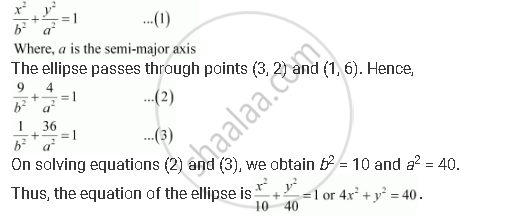Share

# Find the Equation for the Ellipse that Satisfies the Given Conditions: Centre at (0, 0), Major Axis on the Y-axis and Passes Through the Points (3, 2) and (1, 6) - Mathematics

#### Question

Find the equation for the ellipse that satisfies the given conditions: Centre at (0, 0), major axis on the y-axis and passes through the points (3, 2) and (1, 6)

#### Solution

Since the centre is at (0, 0) and the major axis is on the y-axis, the equation of the ellipse will be of the formIs there an error in this question or solution?